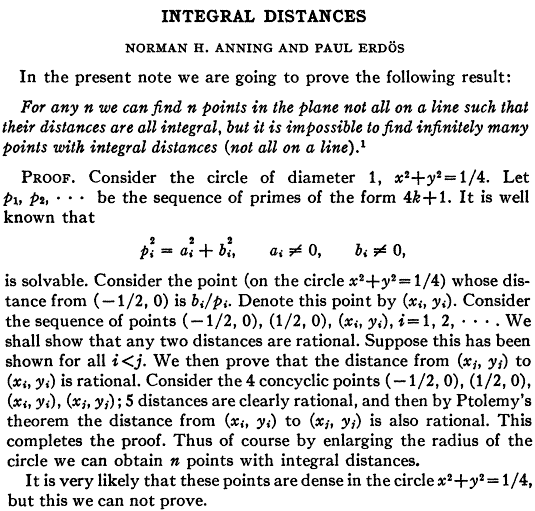# Punti nel piano a distanze reciproche razionali (o intere)

Traduzione e commento del teorema di Erdos-AnningINTEGRAL DISTANCES

NORMAN H. ANNING AND PAUL ERDÖS

In the present note we are going to prove the following result:

For any n we can find n points in the plane not all on a line such that their distances are all integral, but it is impossible to find infinitely many points with integral distances (not all on a line).

PROOF. Consider the circle of diameter 1, x2+y2=1/4.

Let p1, p2, ... be the sequence of primes of the form 4k+1.

It is well known that

pi2 = ai2+bi2, a≠0, b≠0,

is solvable.

Consider the point (on the circle x2+y2=1/4) whose distance from (-1/2, 0) is bi/pi.

Denote this point by (xi, yi).

Consider the sequence of points (-1/2, 0), (1/2, 0), (xi, yi), i=1, 2, ... .

We shall show that any two distances are rational.

Suppose this has been shown for all i<j.

We then prove that the distance from (xj, yj)to (xi, yi)is rational.

Consider the 4 concyclic points (-1/2, 0), (1/2, 0), (xj, yj), (xi, yi); 5 distances are clearly rational, and then by Ptolemy's theorem the distance from (xi, yi), (xj, yj) is also rational.

This completes the proof.

Thus of course by enlarging the radius of the circle we can obtain n points with integral distances.

It is very likely that these points are dense in the circle x2+y2=1/4, but this we can not prove.

Data creazione: marzo 2013

Ultimo aggiornamento: marzo 2013

xhtml 1.1

Sito Web realizzato da Gianfranco Bo